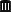## Donald Schuirmann’s opinion [RSABE / ABEL]

Hi BF,

see Donald Schuirmann’spresentation (starting at page 57). For the question whether the CI should include 1 (and his opinion), navigate to page 93.

R-code to reproduce his findings presented on page 94:
library(PowerTOST) # only 1e5 sim's for speed! # (1) pure scaled (without ABE and s-ratio) n        <- seq(12, 48, 12) GMR.min  <- 0.92 swR      <- 0.05 GMR      <- unique(sort(c(seq(GMR.min, 1/GMR.min, length.out=35), 1))) res1     <- data.frame(GMR=GMR) pwr.name <- paste0("pwr.", n) res1[pwr.name] <- NA col      <- c("darkblue", "magenta", "darkgreen", "red") pch      <- c(18, 15, 17, 16) for (j in seq_along(GMR)) {   for (k in seq_along(n)) {     res1[j, pwr.name[k]] <- power.NTIDFDA(theta0=GMR[j], CV=se2CV(swR), n=n[k],                                           details=TRUE)[["p(BE-sABEc)"]]   } } print(res1, row.names=FALSE) op <- par(no.readonly=TRUE) par(mar=c(3, 4, 2, 0) + 0.1) split.screen(figs=c(2, 1)) screen(1) main <- paste0("swR=swT=", swR, "\n\'pure\' NTID RSABE (without ABE and s-ratio)") for (j in seq_along(n)) {   pwr <- res1[[paste0("pwr.", n[j])]]   if (j == 1) {     plot(GMR, pwr, type="n", log="x", ylim=c(0, 1), ylab="power", las=1,          cex.main=0.9, main=main)     abline(h=c(0.05, seq(0, 1, 0.2)), lty=2, col="grey50")     abline(v=1, lty=2, col="grey50")   }   points(GMR, pwr, col=col[j], pch=pch[j], cex=1.15)   lines(GMR, pwr, col=col[j], lwd=2) } legend("bottomright", legend=c(paste("n =", n)), col=col, pch=pch,        pt.cex=1.15, lwd=2, bg="white") # (2) NTID-RSABE (with ABE and s-ratio) res2     <- data.frame(GMR=GMR) pwr.name <- paste0("pwr.", n) res2[pwr.name] <- NA for (j in seq_along(GMR)) {   for (k in seq_along(n)) {     res2[j, pwr.name[k]] <- power.NTIDFDA(theta0=GMR[j], CV=se2CV(swR), n=n[k],                                           details=TRUE)[["p(BE)"]]   } } print(res2, row.names=FALSE) screen(2) main <- paste0("swR=swT=", swR, "\nNTID RSABE (with ABE and s-ratio)") for (j in seq_along(n)) {   pwr <- res2[[paste0("pwr.", n[j])]]   if (j == 1) {     plot(GMR, pwr, type="n", log="x", ylim=c(0, 1), ylab="power", las=1,          cex.main=0.9, main=main)     abline(h=c(0.05, seq(0, 1, 0.2)), lty=2, col="grey50")     abline(v=1, lty=2, col="grey50")   }   points(GMR, pwr, col=col[j], pch=pch[j], cex=1.15)   lines(GMR, pwr, col=col[j], lwd=2) } legend("bottomright", legend=c(paste("n =", n)), col=col, pch=pch,        pt.cex=1.15, lwd=2, bg="white") close.screen(all=TRUE) par(op)

❝ The point is, at Swr of .22, if you pass criteria 1, you generally pass criteria 2. Criteria 3 passes.

Yep. Run the code with
GMR.min <- 100/112 swR     <- 0.22

Dif-tor heh smusma 🖖🏼 Довге життя Україна!Helmut SchützThe quality of responses received is directly proportional to the quality of the question asked. 🚮
Science QuotesIng. Helmut Schütz